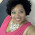## Sunday, 6 October 2013

### Unpaired Student's t-test

If there are two independent datasets that are drawn from a normal distribution, but have the same variance, we can test whether they are different (in particular whether their means are different) using the Unpaired Student's t-test which is a parametric test. Note that a more robust test that is equivalent to this test, and which does not require the assumption of Normality, is the non-parametric Mann-Whitney U test (see blog posting dated 02/09/2013).

Let $N_1$ be the number of samples in the first dataset (with samples $x_1(1),x_1(2),...,x_1(N_1)$), and $N_2$ be the number of samples in the second dataset (with samples $x_2(1),x_2(2),...,x_2(N_2)$).

We calculate the mean of the first dataset, $\mu_1$ as follows
$\Large \mu_1=\frac{1}{N_1}\sum_{n=1}^{N_1}x_1(n)$

We calculate the mean of the second dataset, $\mu_2$ as follows
$\Large \mu_2=\frac{1}{N_2}\sum_{n=1}^{N_2}x_2(n)$

The variance for the first dataset is calculated as follows
$\Large \sigma_1^2=\frac{1}{N_1-1}\sum_{n=1}^{N_1}(x_1(n)-\mu_1)^2$

The variance for the second dataset is calculated as follows
$\Large \sigma_2^2=\frac{1}{N_2-1}\sum_{n=1}^{N_2}(x_2(n)-\mu_2)^2$

Given the means and variances of the two datasets, the $t$ value is derived as follows

$\Large t=\frac{\mu_1-\mu_2}{\sqrt{\frac{1}{N_1}+\frac{1}{N_2}}\sqrt{\frac{\sigma_1^2(N_1-1)+\sigma_2^2(N_2-1)}{N_1+N_2-2}}}$

The overall standard deviation is given by

$\Large \sqrt{\frac{\sigma_1^2(N_1-1)+\sigma_2^2(N_2-1)}{N_1+N_2-2}}$

Once the $t$ value is calculated, the $p$ value (for a two tailed test) is evaluated as follows:-

If $t > 0$,
$p= 2 \times (1 - student\_t\_cdf(t,N_1+N_2-2))$

where $student\_t\_cdf(t,N_1+N_2-2)$ is the Cumulative Distribution Function of the Student's-t probability distribution, with degree of freedom $N_1+N_2-2$ and integrating from $-\infty$ to $t$. The CDF is the following integral, which is evaluated using numerical methods (usually read off a table - if you have an iPhone, you can always download my free app SciStatCalc to evaluate the CDF!)

$\Large \int_{-\infty}^t\frac{\Gamma(\frac{N_1+N_2-1}{2})}{\sqrt{(N_1+N_2-2)(\pi)}\Gamma(\frac{N_1+N_2-2}{2})}(1+\frac{x^2}{N_1+N_2-2})^{-\frac{N_1+N_2-1}{2}}$

If $t<0$
$p= 2 \times (student\_t\_cdf(t,N_1+N_2-2))$

We can think of the $p$ value as the probability that the differences in means between the two datasets are due to chance alone, and that the means are indeed similar (this is our Null Hypothesis). The lower this value, the less likely it is that the difference is due to chance alone, and the more significant the result. The more significant the result is, the more likely that the means of the two datasets are different. We arbitrarily apply some threshold value (the significance value), such as 0.05 (5%), and if the calculated value of $p$ is less than this value, we consider the result to be significant, and reject the Null Hypothesis.

For the two-tailed test, and a significance level of 5%, we can obtain the range of values which covers the 95% confidence level, where the lower limit is

$low\_limit = (\mu_1 - \mu_2) - inv\_t\_cdf(0.975,N_1+N_2-2) \times \sqrt{\frac{1}{N_1}+\frac{1}{N_2}}\sqrt{\frac{(N_1-1)\sigma_1^2 + (N_2-1)\sigma_2^2}{N_1+N_2-2}}$

and the upper limit is

$upp\_limit = (\mu_1 - \mu_2)+inv\_t\_cdf(0.975,N_1+N_2-2) \times \sqrt{\frac{1}{N_1}+\frac{1}{N_2}}\sqrt{\frac{(N_1-1)\sigma_1^2 + (N_2-1)\sigma_2^2}{N_1+N_2-2}}$

the function $inv\_t\_cdf$ is the inverse Student t CDF or quantile function - as the test is two-tailed, for a 5% level, each of the tail will be 2.5% (0.025), so that the quantile is calculated for 1-0.025=0.975.

Datasets with unequal variance

It is worth noting that when the variances of the two datasets are different (which is rarely used in practice, but nevertheless could apply to certain situations), we use Welch's test, where the $t$ value is given by

$\Large t=\frac{\mu_1-\mu_2}{\sqrt{\frac{\sigma_1^2}{N_1}+\frac{\sigma_2^2}{N_2}}}$

The degree of freedom ($df$) for Welch's test is
$\Large df=\frac{(\frac{\sigma_1^2}{N_1}+\frac{\sigma_2^2}{N_2})^2}{\frac{\sigma_1^4}{N_1^2(N_1-1)}+\frac{\sigma_2^4}{N_2^2(N_2-1)}}$

Substituting the $t$ and degree of freedom $df$ values into the Student-t CDF  will yield the desired $p$ value.

Worked Example

Consider two datasets, comprising 4 and 5 samples respectively.

Dataset 1: 1,2,3,4
Dataset 2: 6,7,7,8,9

The number of degrees of freedom is $4+5-2=7$.

The mean and variance of the first dataset is
$\large \mu_1=\frac{1}{4}(1+2+3+4)=2.5$
$\large \sigma_1^2=\frac{1}{3}((1-2.5)^2+(2-2.5)^2+(3-2.5)^2+(4-2.5)^2)=1.667$

The mean and variance of the second dataset is

$\large \mu_2=\frac{1}{5}(6+7+7+8+9)=7.4$
$\large \sigma_2^2=\frac{1}{4}((6-7.4)^2+(7-7.4)^2+(7-7.4)^2+(8-7.4)^2+(9-7.4)^2)=1.3$

We can calculate the $t$ value as follows
$\Large t=\frac{\mu_1-\mu_2}{\sqrt{\frac{1}{N_1}+\frac{1}{N_2}}\sqrt{\frac{\sigma_1^2(N_1-1)+\sigma_2^2(N_2-1)}{N_1+N_2-2}}}=\frac{2.5-7.4}{\sqrt{0.25 + 0.2}\sqrt{\frac{(1.667)(3)+(1.3)(4)}{4+5-2}}}=-6.0512$

The overall standard deviation is $\large \sqrt{\frac{(1.667)(3)+(1.3)(4)}{4+5-2}}$, which is $1.20712$.

The denominator of $t$ is thus $\large 1.20712\times \sqrt{0.45}=0.80976$ - this will be used to evaluate the lower and upper limit of the confidence interval.

Given the $t$ value, the $p$ value (using an appropriate software package) is found
$p= 2 \times (student\_t\_cdf(t,N_1+N_2-2))= 2 \times (student\_t\_cdf(-6.051,7))=0.000515$

Thus, we obtain a very low value of $p$ indicating that the result is very significant. This is not really surprising, when you look at the datasets - the second dataset is much larger than the first, to the extent that there is no overlap.

To calculate the 95% confidence interval, we first need to find the quantile $inv\_t\_cdf(0.975,7)$ using an appropriate table or statistical software - using Octave's tinv() function, we obtain $2.3646$. Thus the lower limit is $2.5-7.4-(2.3646\times 0.80976)=-6.815$, and the upper limit is $2.5-7.4+(2.3646\times 0.80976)=-2.985$.

1.1.2.